• 一元回归
2022-07-25 18:08:18

#pic_center =400x
系列文章：

# 一元回归

## 人工实现代码

# 一元线性回归
import pandas as pd
import numpy as np
import matplotlib.pyplot as plt
from scipy import stats

x = np.array([100, 110, 120, 130, 140, 150, 160, 170, 180, 190])
y = np.array([45, 51, 54, 61, 66, 70, 74, 78, 85, 89])

a, b = 0,0;
def fitted_line(x):
return a*x+b

def linear_regression(x, y, cl=0.9):
"""
linear regression函数y = ax + b
cl为置信水平
alpha为显著性水平
"""
data = pd.DataFrame({'x': x, 'y': y}).sort_values(by='x')
x = np.asarray(data['x'])
y = np.asarray(data['y'])

alpha = 1 - cl
print('置信水平: {}% C.L.\n'.format(100 * cl))
print('显著性水平alpha: {}\n'.format(alpha))

a, b = np.polyfit(x, y, deg=1)

print('斜率: {}\n'.format(a))

print('截距: {}\n'.format(b))

Sxx = np.sum((x - np.mean(x)) ** 2)
Syy = np.sum((y - np.mean(y)) ** 2)
Sxy = np.sum((x - np.mean(x)) * (y - np.mean(y)))

dof = len(x) - 2
print('自由度: {}\n'.format(dof))

sigma = np.sqrt((Syy - a * Sxy) / dof)
print('sigma的无偏估计: {}\n'.format(sigma))

R_sq = a * Sxy / Syy
print('拟合优度R² : {}\n'.format(R_sq))

t_value = stats.t.isf(alpha / 2, dof)
print('t值: {}\n'.format(t_value))

if np.abs(a) / sigma * np.sqrt(Sxx) >= t_value:
print('t检验: 线性回归效果显著\n')
else:
print('t检验: 线性回归效果不显著\n')

print('斜率的置信区间: {}% C.L.\n[{}, {}]\n'.format(
100 * cl,
a - t_value * sigma / np.sqrt(Sxx),
a + t_value * sigma / np.sqrt(Sxx)))

print('截距的置信区间: {}% C.L.\n[{}, {}]\n'.format(
100 * cl,
b - t_value * sigma * np.sqrt(1. / len(x) + np.mean(x) ** 2 / Sxx),
b + t_value * sigma * np.sqrt(1. / len(x) + np.mean(x) ** 2 / Sxx)))

print('回归函数的函数值的点估计和置信区间: {}% C.L.\n下区间端点: {}\n上区间端点: {}\n'.format(100 * cl,
fitted_line(x) - t_value * sigma * np.sqrt(
1. / len(x) + (x - np.mean(x)) ** 2 / Sxx),
fitted_line(x) + t_value * sigma * np.sqrt(
1. / len(x) + (x - np.mean(x)) ** 2 / Sxx)))

print('观测值的点预测和预测区间: {}% C.L.\n下区间端点: {}\n上区间端点: {}\n'.format(100 * cl,
fitted_line(x) - t_value * sigma * np.sqrt(
1. + 1. / len(x) + (x - np.mean(x)) ** 2 / Sxx),
fitted_line(x) + t_value * sigma * np.sqrt(
1. + 1. / len(x) + (x - np.mean(x)) ** 2 / Sxx)))

fig = plt.figure()
ax.scatter(x, y, s=1, c='k', )
ax.plot(x, fitted_line(x), lw=1, label='Linear regression')
ax.fill_between(x,
fitted_line(x) - t_value * sigma * np.sqrt(1. / len(x) + (x - np.mean(x)) ** 2 / Sxx),
fitted_line(x) + t_value * sigma * np.sqrt(1. / len(x) + (x - np.mean(x)) ** 2 / Sxx),
alpha=0.3,
label=r'Confidence limit'.format(100 * cl))
ax.set_xlabel('$x$')
ax.set_ylabel('$y$')
ax.legend()
fig.savefig('Linear regression.png', dpi=300)

linear_regression(x,y)


Python实现一元线性回归

## statsmodels实现

import csv
import sys

import matplotlib.pyplot as  plt
import numpy
import numpy as np
import statsmodels.api as  sm
import xlrd
import openpyxl
import xlsxwriter
# xlrd读取表格数据，支持xlsx和xls格式的excel表格；xlwt写入excel表格数据

file_path = "E:\\code\\experiment\\data\\singleRegression\\source\\userId\\testId\\source.xlsx"
target_path = "E:\\code\\experiment\\data\\singleRegression\\result\\userId\\testId"
variable = "自变量"
dep_variable = "因变量"

# Matplotlib 默认情况不支持中文，我们可以使用以下简单的方法来解决：
plt.rcParams['font.sans-serif'] = ['SimHei']
plt.rcParams['axes.unicode_minus'] = False

def get_excel_data_all(file_path):
data_excel = xlrd.open_workbook(file_path)
table = data_excel.sheets()  # 通过索引顺序获取sheet
row_count= table.nrows  # 获取该sheet中的有效行数
col_count = table.ncols  # 获取该sheet中的有效列数
result= []
for i in range(row_count):
result.append(table.row_values(i, start_colx=0, end_colx=None))
row_data = table.row_values(0, start_colx=0, end_colx=None)
return row_count, col_count, result

if __name__ == '__main__':
for i in range(0, len(sys.argv)):
pass
# print(i,"---" , sys.argv[i])
if len(sys.argv)>1:
file_path = sys.argv
if len(sys.argv) > 2:
target_path = sys.argv
if len(sys.argv)>4:
variable = sys.argv
dep_variable = sys.argv
row_count, col_count, result = get_excel_data_all(file_path)
# 一元回归只能有1个变量
if col_count > 2:
col_count = 2
result = numpy.array(result)
x_data = []
y_data = []
for i in range(1, row_count):
for j in range(0, col_count - 1):
x_data.append(float(result[i][j]))
y_data.append(float(result[i][col_count - 1]))

model = sm.OLS( y_data,x_data)
res = model.fit()
beta = res.params
print(res.params)  # # 取系数
summary = res.summary()
print(res.summary())  # # 回归 分析摘要
with open(target_path+"\\result.txt",
"w",encoding="utf-8") as f:
f.write(str(summary))
Y = res.fittedvalues  # 预测值
# 原始数据
plt.scatter(x_data,y_data, marker = "o", s = 5,  cmap="viridis", alpha=0.3, label="原数据")
# 预测数据
plt.plot(x_data, Y, 'r--.', label='预测数据')
plt.legend(loc='upper left')  # 图例，显示labe
plt.xlabel(variable)
plt.ylabel(dep_variable);
plt.savefig(target_path+'\\result.png' )

# with open("E:\\code\\experiment\\back\\formal\\data\\data_result\\single_regression\\x.csv",
#           "a",encoding="utf-8") as f:
#     writer = csv.writer(f)
# writer.writerow(row)
# f.write(pic_target_path+'\\2.png')

# plt.plot(x_data,Y,color="r", linestyle="solid",linewidth=2,marker="o")
plt.show()


### sklearn.linear_model

import csv
import sys
import pandas as pd

import numpy as np

import matplotlib.pyplot as plt

from sklearn.linear_model import LinearRegression

from sklearn.metrics import r2_score

import statsmodels.api as sm

# xlrd读取表格数据，支持xlsx和xls格式的excel表格；xlwt写入excel表格数据

file_path = "E:\\code\\experiment\\data\\singleRegression\\source\\userId\\testId\\source.xlsx"
target_path = "E:\\code\\experiment\\data\\singleRegression\\result\\userId\\testId"
variable = "年龄"
dep_variable = "身高"

# Matplotlib 默认情况不支持中文，我们可以使用以下简单的方法来解决：
plt.rcParams['font.sans-serif'] = ['SimHei']
plt.rcParams['axes.unicode_minus'] = False

if __name__ == '__main__':
for i in range(0, len(sys.argv)):
pass
# print(i,"---" , sys.argv[i])
if len(sys.argv)>1:
file_path = sys.argv
if len(sys.argv) > 2:
target_path = sys.argv
if len(sys.argv)>4:
variable = sys.argv
dep_variable = sys.argv

# 查看数据时，输入：
# plt.figure(figsize=(16, 8))
x_data = data[['年龄']]
y_data = data[['身高']]
# “未命名：0”列是多余的。所以，我们把这一列删除。
# data.drop(['Unnamed: 0'], axis=1)
# plt.scatter(data.loc[:, variable], data.loc[:, dep_variable])

# 画出散点图，求x和y的相关系数
model = LinearRegression()
res =  model.fit(x_data,y_data)
# 预测数据
predictions = model.predict(x_data)
# 原始数据
plt.scatter(x_data, y_data, marker="o",   label="原数据")

plt.plot(x_data, predictions, 'r--.',linewidth=3, label='预测数据')
plt.title("Y = {:.5} + {:.5}X".format(model.intercept_, model.coef_))
# plt.show()
plt.legend(loc='upper left')  # 图例，显示labe
plt.xlabel(variable)
plt.ylabel(dep_variable)

plt.savefig(target_path + '\\result.png')

print("The linear model is: Y = {:.5} + {:.5}X".format(model.intercept_, model.coef_))

est = sm.OLS(y_data,x2).fit()
summary = est.summary()
print(est.summary())

with open(target_path+"\\result.txt",
"w",encoding="utf-8") as f:
f.write(str("The linear model is: Y = {:.5} + {:.5}X".format(model.intercept_, model.coef_)))
f.write("\n")
f.write("==============================================================================\n")
f.write(str(summary))

plt.show()


## 报告注解

            content.append(Graphs.draw_text('Dep. Variable: 响应变量的名称， Dep为Depended的缩写'
'Model/Method: 表示这里使用了普通最小二乘法OLS'
'Date/Time: 对模型进行估计的日期和时间'
'No. Observations: 样本容量'
'Df Residuals: 样本容量减去参与估计的参数个数'
'Df Model:用到的解释变量的个数(不是参数个数)'
'Covariance Type:协方差类型，默认为nonrobust'
'F-statistic/Prob (F-statistic):方差分析结果'
'Log-Likelihood:最大似然对数'
'AIC:赤池信息准则'
'BIC:贝叶斯信息准则，属于信息准则的一种'))


## 数据读取

### 无表头，列名 .csv格式value_array = np.genfromtxt("./datasets/test.csv", delimiter=',')
print(value_array)
x = value_array
y = value_array
print(x)
print(type(x))
print(y)


python数据分析：一元线性回归

### panda读取csv

from pandas import read_csv
from matplotlib import pyplot as plt
from sklearn.linear_model import LinearRegression
#画出散点图，求x和y的相关系数
plt.scatter(data.loc[:,'广告投入'], data.loc[:,'销售额'])
Model = LinearRegression()
x = data[['广告投入']]
y = data[['销售额']]



python去读csv文件，以及numpy的ndarray与pandas的series和dataframe之间互转

### panda读取excel

    data = pd.read_excel(file_path)
# 画出散点图，求x和y的相关系数
plt.scatter(data.loc[:, '年龄'], data.loc[:, '身高'])
x_data = data[['年龄']]
y_data = data[['身高']]


### panda多元回归

import csv
import math
import sys
import pandas as pd
import numpy as np
import matplotlib.pyplot as plt
from sklearn.linear_model import LinearRegression
from mpl_toolkits.mplot3d import Axes3D
import matplotlib as mpl
import statsmodels.api as sm
# xlrd读取表格数据，支持xlsx和xls格式的excel表格；xlwt写入excel表格数据
file_path = "C:\\Users\\ytm\\Desktop\\test.xlsx"
target_path = "C:\\Users\\ytm\\Desktop\\result\\multi"
variable = ["id","age","number"]
dep_variable = "score"

# Matplotlib 默认情况不支持中文，我们可以使用以下简单的方法来解决：
plt.rcParams['font.sans-serif'] = ['SimHei']
plt.rcParams['axes.unicode_minus'] = False

# 0 program
# 1 fileCompleteFileName
# 2   targetDir
# 3-  variable
# -1  depVariable

if __name__ == '__main__':
args_len =  len(sys.argv)
for i in range(0, args_len):
print(i,"---" , sys.argv[i])
if args_len > 1:
file_path = sys.argv
if args_len > 2:
target_path = sys.argv
dep_variable = sys.argv[-1]
variable = []
for j in range(3, args_len-1):
variable.append(sys.argv[j])

x_data = data[[i for i in variable]]

y_data = data[[dep_variable]]
x = np.column_stack(x_data)

model = LinearRegression()
res =  model.fit(x_data,y_data)

resultForm = "The linear model is: Y = {:.5} ".format(model.intercept_)
for i  in  range(0,len(variable)):
resultForm += "+ ({:.5}*".format(model.coef_[i])+variable[i] + ")  "
resultForm += "\n\n\n"

# 评估模型

est = sm.OLS(y_data,X2)
est2 = est.fit()
summary = est2.summary();
print(est2.summary())
with open(target_path+"\\result.csv",
"w",encoding="utf-8") as f:
for i in range(0, len(variable)):
resultForm += "+ ({:.5}*".format(model.coef_[i]) + variable[i] + ")  "
resultForm += "\n\n\n"
f.write(resultForm)
f.write("==============================================================================\n")
f.write(str(summary))

# mpl.rcParams['legend.fontsize'] = 10

x_data = [ x_data[i].values for i in variable]
y_data = y_data[dep_variable].values

min_x = np.array([ x_data[i].min() for i in range(0,len(variable))])
max_x = np.array([x_data[i].max() for i in range(0, len(variable))])
step_x = (max_x - min_x+1)/5
min_y = y_data.min()
max_y = y_data.max()
step_y = math.ceil((max_y-min_y+1)/5)

for i in range(0,len(variable)):
for j in range(i+1, len(variable)):
fig = plt.figure()
# 坐标轴标签重叠
plt.tight_layout()
ax.scatter(x_data[i], x_data[j], y_data)
ax.set_xticks(np.arange(min_x[i], max_x[i]+step_x[i], math.ceil(step_x[i])))
ax.set_xlabel("x:"+variable[i])
ax.set_yticks(np.arange(min_x[j], max_x[j]+step_x[j], math.ceil(step_x[j])))

ax.set_ylabel("y:"+variable[j])
# ax.set_yticks([np.linspace(minx-1, maxx+1, (maxx-minx + 1)/10)])
print("y:"+variable[j])
ax.set_zticks(np.arange(min_y, max_y+step_y, step_y))
ax.set_zlabel("z:"+dep_variable)
plt.savefig(target_path+"\\result-"+str(i)+"-"+ str(j)+".png")



# 多元回归

statsmodel官方

python statsmodel 回归结果提取（R方 T值 P-value)

## JMP借鉴Scipy 显著性检验

多元线性回归模型可视化python 机器学习
更多相关内容
• 关于一元回归分析和多元回归分析的matlab代码，含有测试数据，直接就可以运行，然后出图，注释清楚，通俗易懂，每一步都有注释，比较详细。
• 多元线性回归 b=regress( Y, X ) 1确定回归系数的点估计值 统计工具箱中的回归分析命令 对一元线性回归取p=1即可 3画出残差及其置信区间 rcoplotrrint 2求回归系数的点估计和区间估计并检验回归模型 [b, bint,r,rint...
• 包括基于矩阵运算计算回归系数并添加趋势线，基于多项式拟合计算回归系数并添加趋势线，借助回归分析程序包计算回归系数并估计检验统计量，及预测和各统计量。
• 介绍回归分析的一些基础知识，并介绍了一元、多元回归验证。一元回归
• 前言 由于时间关系，本节内容简略。 案例分析求解 问题一 首先，先看第一个例子，众所周知，车辆都是具有使用寿命和使用年限的，一般来说，随着使用时间的增长，车辆的新度（几成新）也在不断地降低，车辆此时...

目录

一、前言

二、案例分析求解

2.1问题一

2.1.1分析

2.1.2Matlab求解一元线性回归模型

2.1.3结果

2.2问题二

2.2.1分析

2.2.2Matlab求解

2.2.3结果

三、回归分析

# 一、前言

对于线性回归方程模型，在数据点拟合中，常常利用多项式进行拟合，找出一条数据点与线上距离平方和最小的曲线。

1.[p,s]=polyfit(x,y,n)为进行x向量与y向量进行多项式求解的函数，n为拟合函数的次数。p为由高到低的系数矩阵，s为结构数组。

2.ployval(p,x)为求拟合方程p在x处的值。

3.r=corrcoef(x,y)为求相关系数矩阵，1表示最大程度的正相关，-1表示最大程度的负相关。

# 二、案例分析求解

## 2.1问题一

首先，先看第一个例子，众所周知，车辆都是具有使用寿命和使用年限的，一般来说，随着使用时间的增长，车辆的新度（几成新）也在不断地降低，车辆此时的市场价格也在发生着变化。请研究使用时长(x)与车辆市场价格(y)之间的关系。

## 2.1.1分析

对于使用时长和市场价格之间的关系，首先应利用plot绘图函数进行数据点的刻画，结合所学知识进行初步判断，进行多项式拟合，并验证设想。

x=1:10;

y=2650 1942 1493 1056 766 539 485 291 224 202;

## 2.1.2Matlab求解一元线性回归模型

1.利用软件进行数据点的刻画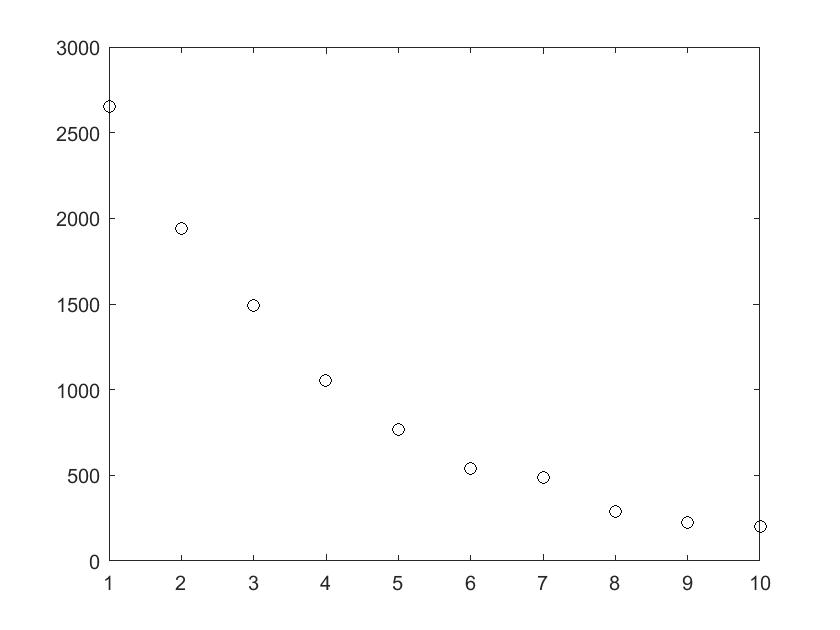结合所学知识，观察图像可知，数据点的解读为，随着使用年限的增加，车辆的时长价格又迅速的下降趋势转变为缓慢的下降趋势。

相关程序：

clear
x=1:10;
y=[2650 1942 1493 1056 766 539 485 291 224 202];
plot(x,y,'ok')

2.图像呈现的是指数函数图像，则令,验证x与z是否为线性关系，以映证设想。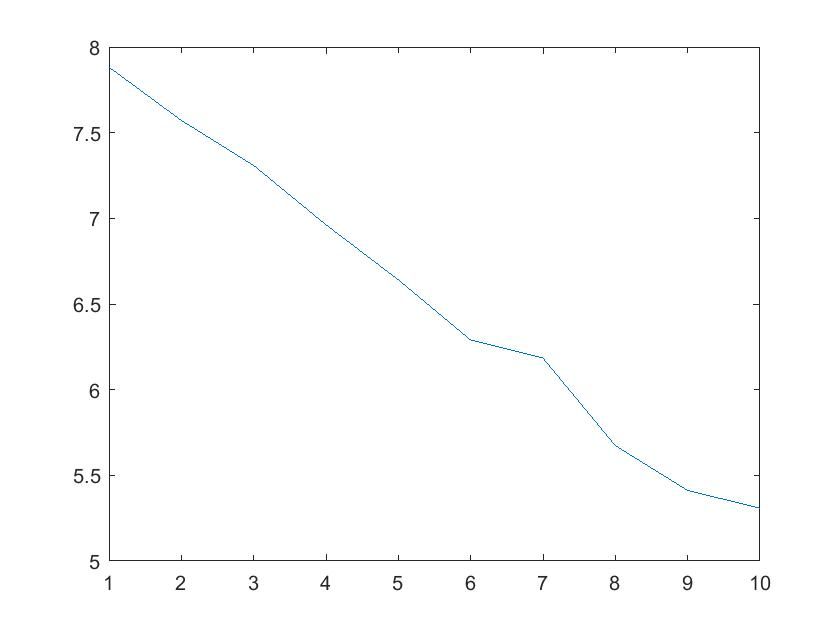经过上图可知，我们可以发现除个别点以外，基本呈线性关系。

相关代码：

clear
x=1:10;
y=[2650 1942 1493 1056 766 539 485 291 224 202];
z=zeros(size(y))
N=length(y);
for i=1:N
z(i)=log(y(i))
end
plot(x,z)


3.假设这条直线的方程为，利用plotfit函数求解相应的a，b值 。

相关代码

clear
x=1:10;
y=[2650 1942 1493 1056 766 539 485 291 224 202];
z=zeros(size(y))
N=length(y);
for i=1:N
z(i)=log(y(i))
end
[p,s]=polyfit(x,z,1)


## 2.1.3结果

p =
-0.2979    8.1615
s =
R: [2x2 double]
df: 8
normr: 0.2311

根据求解结果可知，一元线性回归方程为.其中，df为自由度，normr为拟合误差平方和的算数平方根。

# 2.2问题二

想不到吧，上次从这里断了我还会回来，说实话，我不是断更鸟。继续...

多元线性回归问题在我的生命里说实话占据很大的色彩，回归和拟合不一样，这是我查到的资料，具体怎么不一样，细细听我讲。拟合是什么呢，就是给出你两串数据点，plot函数可视化之后，用一条光滑的曲线连接起来，因为这条曲线有无数种可能（比如两个点用曲线连接，肯定有无数种可能），从而有各种拟合方法。常用的有最小二乘法拟合，也可以用polyfit多项式拟合。

拟合、插值、逼近区别：拟合是已知点列，从整体上靠近它们；插值是已知点列且完全经过点列；逼近是已知曲线或点列，通过逼近使得构造的函数无限靠近它们。

回归是什么，就是有很多自变量，很多因变量，现在要研究它们之间的关系，是一种统计分析方法，一元线性回归就是一个自变量与一个因变量之间的关系，自变量个数大于1个就是多元回归，因变量个数大于1个称为多重回归。

详细见三。

多元线性回归案例：（来源@川川菜鸟）

#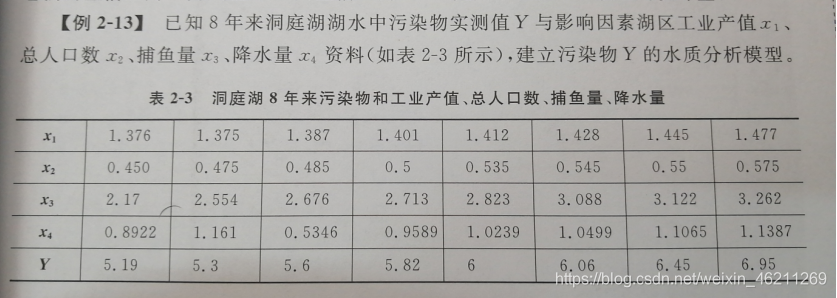## 2.2.1分析

如图，将实际问题转化为数学问题，这也是数学建模过程中比较重要的一步，此问题中，探究湖水污染物实测值与工业产值、总人口数、捕鱼量、降水量之间的关系，数学问题为：因变量Y与自变量x1、x2、x3、x4之间的关系，有1个因变量，4个自变量为多元回归模型，利用Matlab求解。

## 2.2.2Matlab求解

clear
x1=[1.376 1.375 1.387 1.401 1.412 1.428 1.445 1.477];
x2=[0.45 0.475 0.485 0.5 0.535 0.545 0.55 0.575];
x3=[2.17 2.554 2.676 2.713 2.823 3.088 3.122 3.262];
x4=[0.8922 1.161 0.5346 0.9589 1.0239 1.0499 0.1065 1.1387];%输入自变量数据
y=[5.19 5.3 5.6 5.82 6 6.06 6.45 6.95];%输入因变量数据
y=y.';转置，列向量
x=[ones(size(x1.')),x1',x2.',x3.',x4.'];%自变量矩阵，每一列为一个变量
[b,bint,r,rint,stas]=regress(y,x)%多元回归

## 2.2.3结果

b =
-13.5345
12.8735
1.9750
0.1068
-0.0521
bint =
-26.4895   -0.5796
0.1092   25.6378
-16.0651   20.0151
-1.5914    1.8050
-0.4713    0.3671
r =
-0.0635
-0.0170
0.0631
0.0914
0.0523
-0.1403
-0.0318
0.0457
rint =
-0.1413    0.0144
-0.4562    0.4223
-0.3518    0.4781
-0.4043    0.5872
-0.0548    0.1595
-0.3962    0.1156
-0.2800    0.2163
-0.1144    0.2057
stas =
0.9824   41.8666    0.0058    0.0141

则自变量与因变量的关系是.

其中，b代表多项式中次数由低到高的系数，若需要包含常数项，需增加一个由1组成的列。bint代表返回系数估计值为95%置信区间的矩阵，返回由残差组成的向量r，返回矩阵rint，诊断离群值的区间。

# 三、回归分析

续前节，继续讲，回归分析的主要过程：

1.从给定的数据出发，确定某些变量之间的关系式，就是建立把实际问题转化为数学问题，设关系式，利用给定数据求解系数的过程。

2.检验这些关系式的可信任程度。

3.在多个自变量影响一个自变量的关系中，判断自变量的影响是否显著，并将影响显著的自变量引入模型，剔除不显著的变量。逐步回归、向前回归、向后回归。

4.利用求出的关系式对某一过程进行预测或控制。

回归的种类有线性回归、曲线回归、二元logistic回归、多元logistic回归。

在这里提一点啊，记得spss中分析里有个相关与偏相关，可以利用相关找出你的目标变量与相关性大的变量之间的关系，然后进行筛选、剔除，再利用回归进行求解，这是一点点想法。

相关分析研究的是现象之间是否相关、相关的方向和密切程度，一般不区分自变量和因变量。

回归分析是确立自变量与因变量直接的具体表达式，来描述之间的具体关系的。

附：欢迎指正。

最小二乘法会单独写一篇博文。

展开全文• 一元线性回归和二元线性回归的matlab实例编程 包括有多元线性回归模型
• 运用Python的数组和矩阵操作模拟验证一阶自回归模型中，自回归系数OLS估计量的有限样本偏差问题。
• SAS实验四 一元回归分析 棉花红铃虫第一代产卵高峰日百株卵量x（粒）与百株累计卵量y（粒）的8组观测数据如下表 炼钢厂出钢时所用的盛钢水的钢包，在使用过程中由于钢液及炉渣耐火材料的浸蚀，其容积不断增大.经试验...

实验四实验指导书：

## 实验目的和要求

(1)通过上机操作使学生掌握用proc import语句将excel数据导入SAS，生成SAS数据集；（2）掌握用proc reg过程对数据进行一元线性回归分析和可转化一元线性的回归分析；（3）会正确阅读一元回归分析的运行结果，并写出回归方程。

## 实验步骤：

### 1.棉花红铃虫第一代产卵高峰日百株卵量x（粒）与百株累计卵量y（粒）的8组观测数据如下表：

棉花红铃虫第一代卵量的观测数据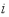1       2       3       4      5       6      7       8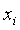14.3     14.0    69.3    22.7    7.3     8.0     1.3     7.9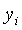46.3     30.7    144.6   69.2    16.0    12.3    2.7     26.3

（1）试建立一元线性回归方程并作回归方程的显著性检验.

代码：

data aaa1;
input i x y@@;
cards;
1 14.3 46.3 2 14.0 30.7 3 69.3 144.6 4 22.7 69.2
5 7.3 16.0 6 8.0 12.3 7 1.3 2.7 8 7.9 26.3
;
run;
proc reg data=ex4.aaa1 outest=outest;
model y=x;
plot R.*P. R.*X  R.*NQQ.;
output out=out p=p R=R student=stdr cookd=cookd;
run;


截图：

一元线性回归方程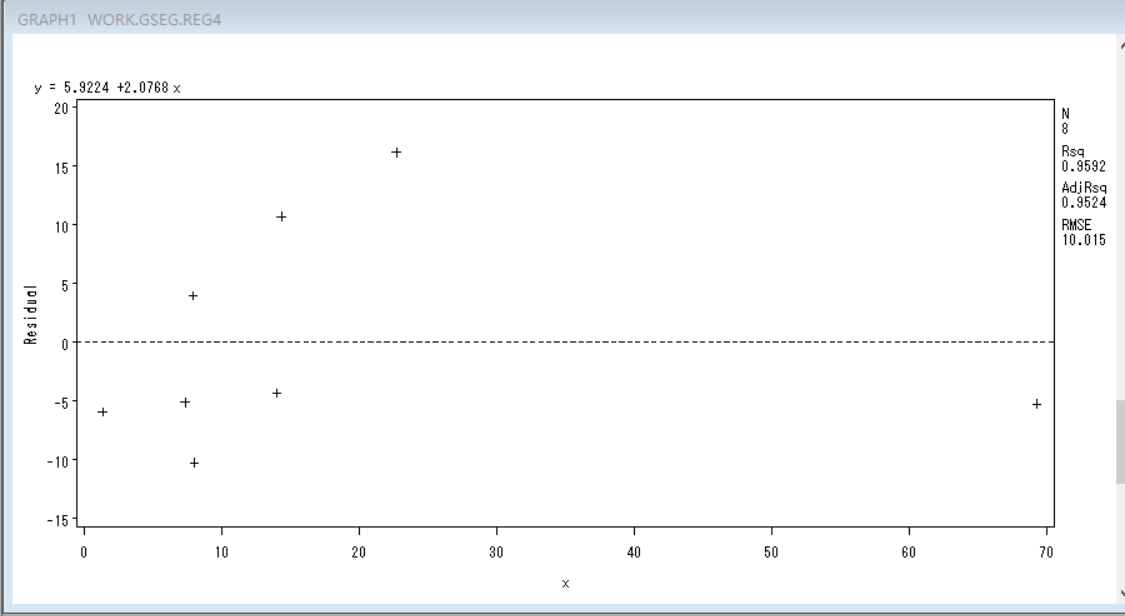回归方程的显著性检验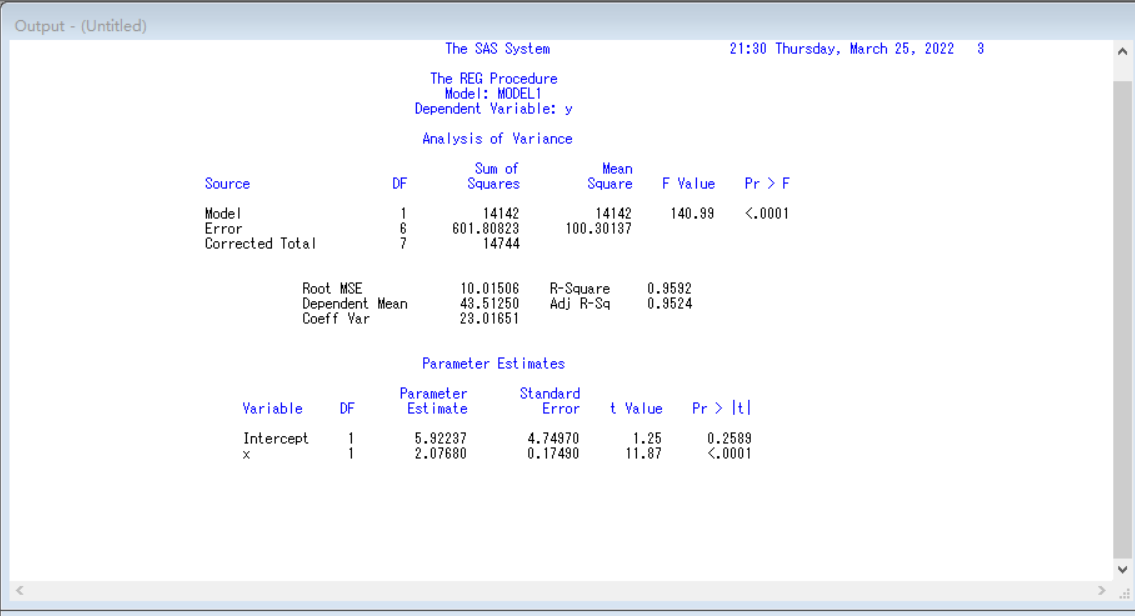（2）如果令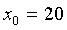，试求此点的预测值及置信水平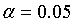的置信区间.

求预测值代码：

data out2;
set outest;
y=intercept+x*20;
keep y;
proc  print data=out2;
run;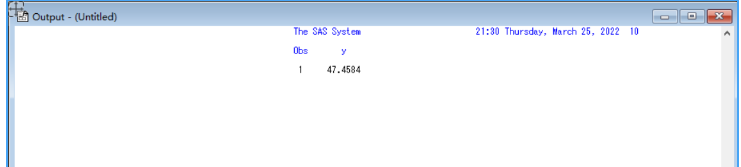当X=20时，y的预测值是47.4584

求置信区间代码

proc means data=aaa1 clm;
run;


截图：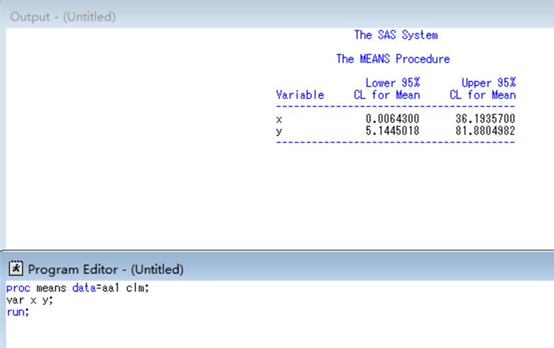### 2.炼钢厂出钢时所用的盛钢水的钢包，在使用过程中由于钢液及炉渣耐火材料的浸蚀，其容积不断增大.经试验得钢包的容积Y与相应使用的次数X的数据如下表

钢包浸蚀数据

 X y X y 2 106.42 11 110.59 3 108.2 14 110.6 4 109.58 15 110.9 5 109.5 16 110.76 7 110 18 111 8 109.93 19 111.2 10 110.49

（1）绘制Y对X的散点图，从图能否看出Y与X的函数关系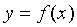将上述的表格导入到excel表中，注意后缀为.xls格式的，否则会导入失败

代码：

proc import out=bbb1
datafile="G:\实验课作业\应用统计方法\实验四\gb.xls"
DBMS=EXCEL2000 replace;
proc plot data=Ex4.bbb1;
plot y*x="+";
run;


截图：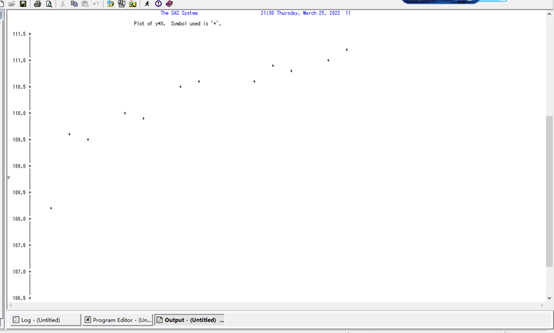（2）假定Y与X的函数关系为①双曲线：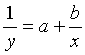,②幂函数：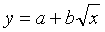，③对数函数：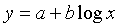,④负指数函数：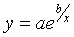，试作变量变换化非线性回归为线性，并用REG过程建立回归方程.

①双曲线：代码：

data bbb2;
set Ex4.bbb1;
x=1/x;
y=1/y;
proc reg;
model y=x;
plot y*X;
run;


截图：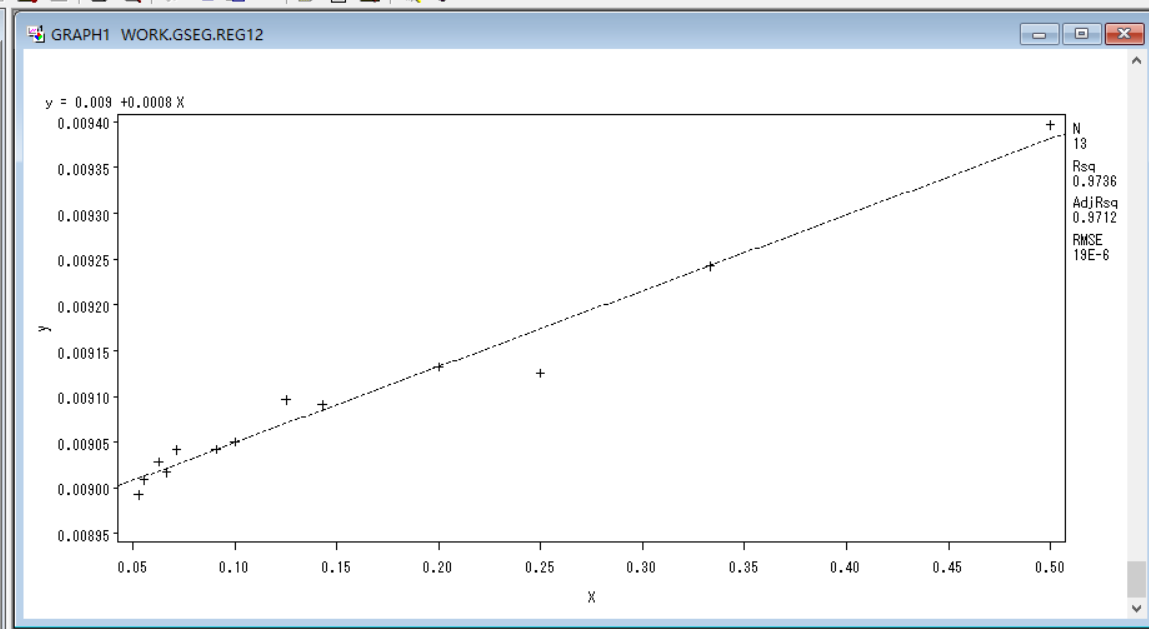②幂函数：代码：

data bbb3;
set Ex4.bbb1;
x=sqrt(x);
y=y;
proc reg;
model y=x;
plot y*X;
run;


截图：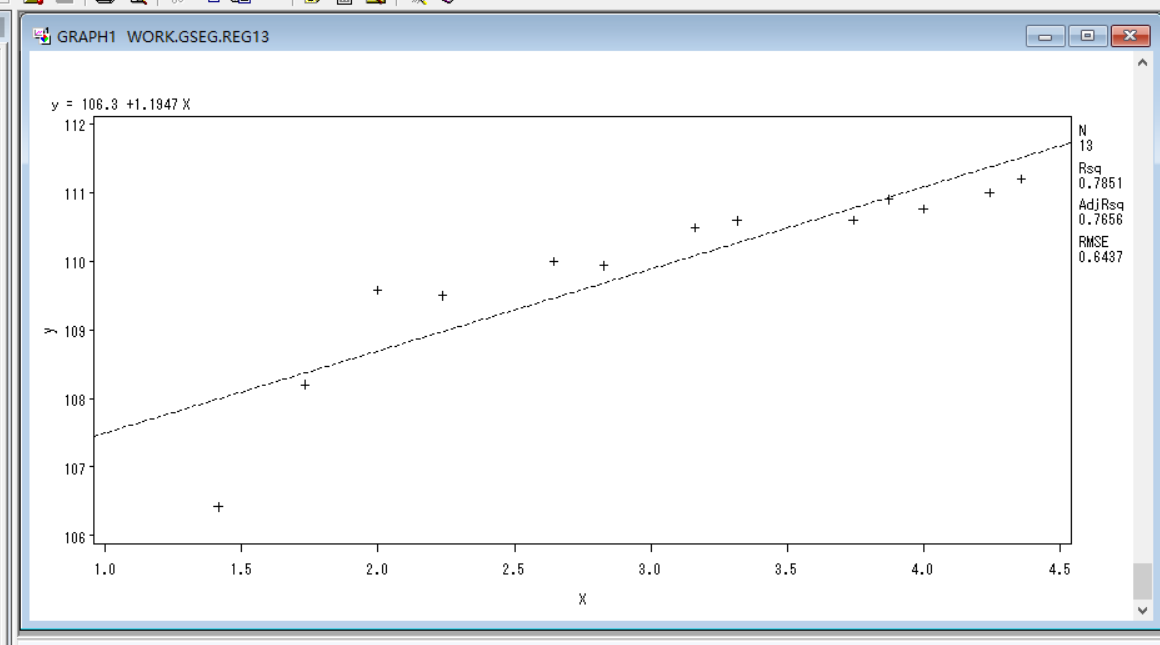③对数函数：代码：

data bbb4;
set Ex4.bbb1;
x=LOG(x);
y=y;
proc reg;
model y=x;
plot y*X;
run;


截图：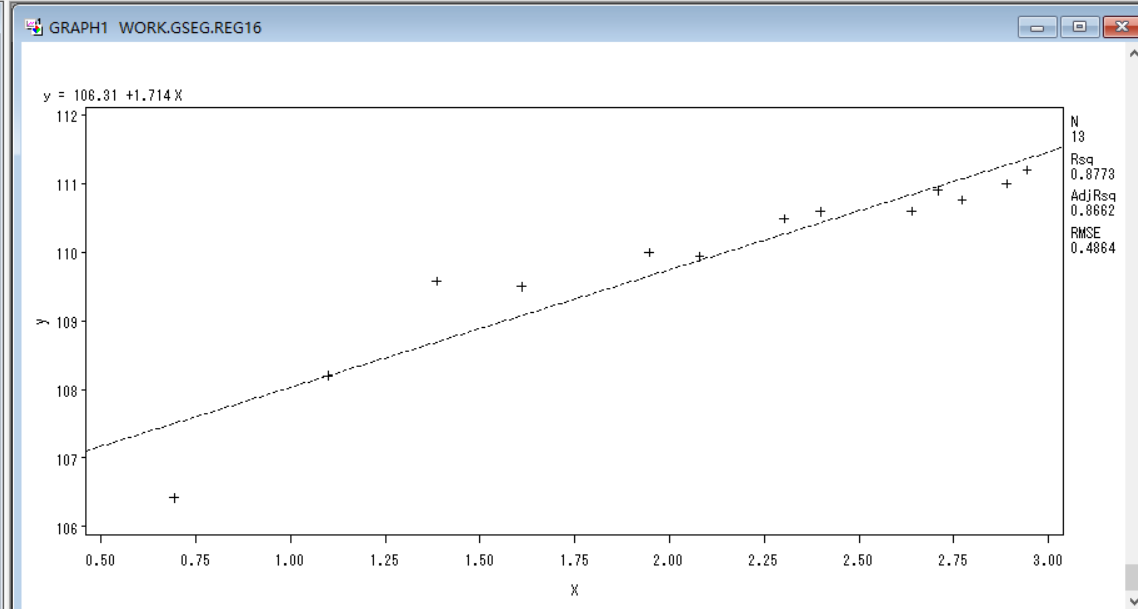④负指数函数：代码：

data bbb5;
set Ex4.bbb1;
x=EXP(1/x);
y=y;
proc reg;
model y=x;
plot y*X;
run;


截图：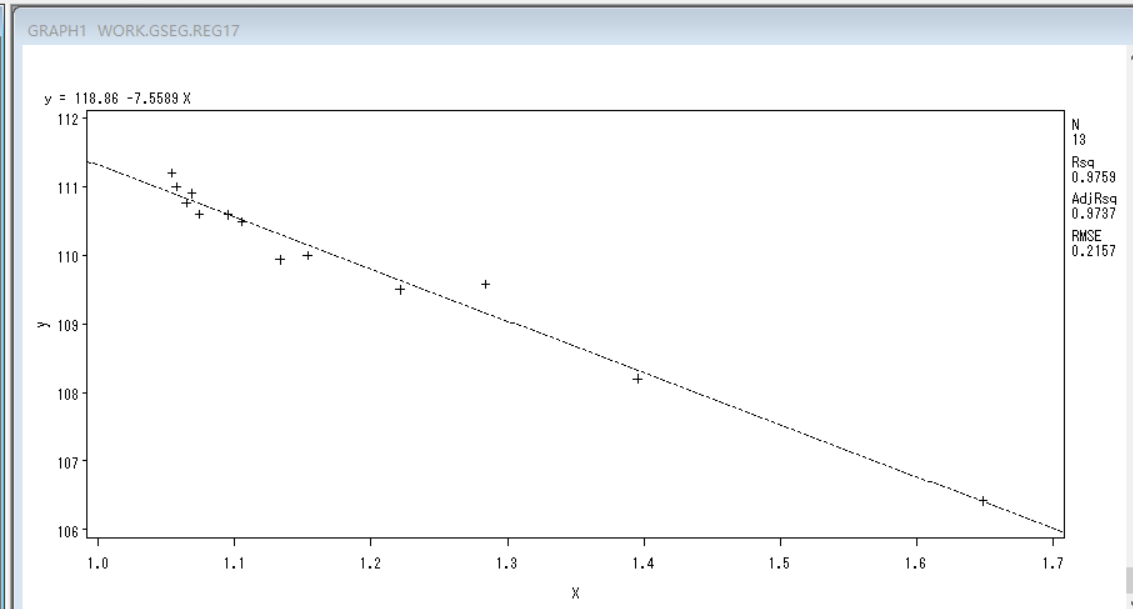（3）比较以上四种函数关系，找出最佳的拟合曲线.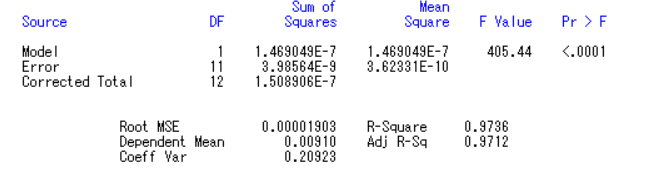②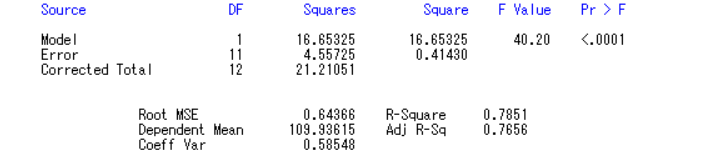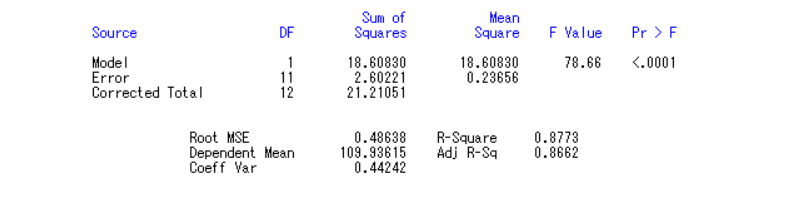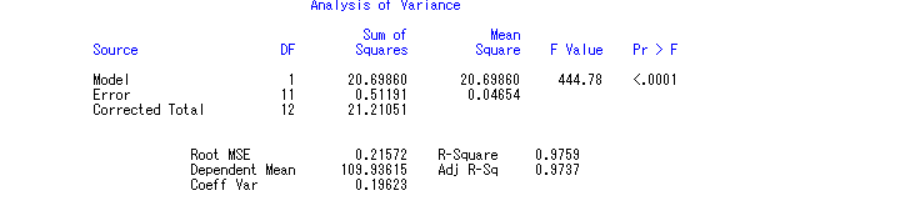通过比对R-Square值（ 就是R的平方，R方通常用来描述数据对模型的拟合程度的好坏），第四个型函数的拟合值最高，所以最佳拟合函数为展开全文• 工程管理作为工程技术与管理交互复合型，而这一份一元回归（Excel模板）则可以帮助更好更快的了解与一元...该文档为一元回归（Excel模板），是一份很不错的参考资料，具有较高参考价值，感兴趣的可以下载看看
• 话不多说开始学习线性回归（线性回归，猛地一听是不是想起了线性回归方程，对的就是你了解的线性回归方程，这里我们说的是一元线性回归方程，不难，你会一次方程就会一元线性回归方程，这里不过只是增加了几个概念...无论时代再如何变，人类的知识体系永远不会变，除非这个世界本身就不是真实的。

很多人不喜欢数学，其实这是普遍现象，但是这个世界就是依靠数学运转着，除非你会魔法，那么你就不需要数学，从入行到现在，我依然不会觉得算法不重要，你只要打好业务代码就好了，难得就不做，这一点我是不行的，就像今天研究dns一样，找了许许多多的方案，最终决定尝试一种方案，一天下来没做啥，真的累了，数据更直观，用相应的公式和数据进行分析，这样就能做出你预期想要的答案，可能有种感觉，回到了中学（如果真能回到中学，我还真想回去，有时候感觉爱因斯坦的物质守恒定论可能并不真确，当然这只属于我个人观点，机器学习的时代的来临为我们能够节约多少时间，这是显而易见，这条路还很长，我们的路还需要慢慢摸索，加入真的有一天人工智能达到了钢铁侠中的jarvis一样，那我真的解放了，世界会怎么样，真的值得期待）。

机器世代的来领早已经开始，下一个时代将是人工智能和数据的时代，也是信息安全保卫战的时代。

信息在那个时代都不过时，我们是信息的使用者，也是信息的创造者，我们这个时代还没有将信息完全的分类和守护好，创造利益是我们当前要做的事，利益的趋向能使当前技术的成熟。

话不多说开始学习线性回归（线性回归，猛地一听是不是想起了线性回归方程，对的就是你了解的线性回归方程，这里我们说的是一元线性回归方程，不难，你会一次方程就会一元线性回归方程，这里不过只是增加了几个概念）：

一元线性回归的基本形式就是。通过这个我们可以得到的一个结论是。这里我们可以用这个方程代替：,这个我想大家就认识了，通常我们是依照下面的图求出方程：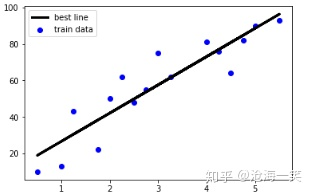那时候们是手动求出这条直线，也就是这个方程。而在实际中我们可以利用简单的一元一次方程进行线性回归预测。

这里我说几个概念：线性相关、协方差、相关系数、决定系数R平方，以及最小二乘法。

线性相关：

这里有几个特性：正向相关、负相关、不相关，这几个特性其实是依靠数据将这些相关性反映出来。

正相关：随着x轴值的增大，y增大，这就是正相关，这里我们讨论的是一次方程，要说正相关其实和系数b相关[也就是斜率],斜率为正，那就是正相关，下图就是正相关的绘制曲线：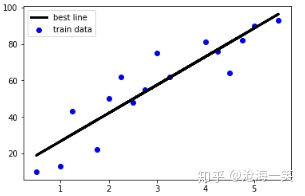负相关与正相关相反的特性就是负相关，随着x值增大，y值减小，最主要的反应就是斜率为负，不难想象散点图的分布：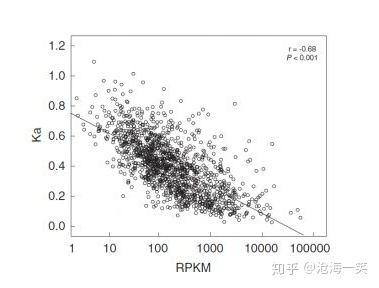无相关（没有相关性）：这个特性就是既不是正相关，又不是负相关，两种相关性都不符合，那么就是无相关（这种说法可能也不准确，理论上说一切事物都能够用数学解释，主要是你需要寻找一种模型解释现有的数据），下图我们不能用线性方程解释：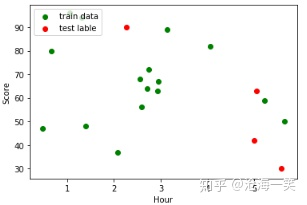协方差（协方差主要描述变量之间的相关性）两个数据点的协方差(：这里其实求得是单一的期望。

协方差也有缺点：当我们利用协方差描述相关性时，如果两个点的数据变化幅度不想同时，所求的期望差值太大，我们就不能够用协方差进行相关性描述，为此我们需要排除这样的影响，这里我们就需要利用相关系数进行相关性描述。

对于协方差的基本了解我推荐看这篇博客：

如何通俗易懂地解释「协方差」与「相关系数」的概念？ - GRAYLAMB的回答 - 知乎 https://www.zhihu.com/question/20852004/answer/134902061

相关系数：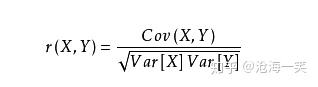相关系数系数能够将协方差的影响剔除掉，这一点我们需要进一步学习：

这里我依然推荐刚才那篇博客：

如何通俗易懂地解释「协方差」与「相关系数」的概念？ - GRAYLAMB的回答 - 知乎 https://www.zhihu.com/question/20852004/answer/134902061

这里我们可以用一句话总结：相关系数是标准化后的协方差

相关系数有三个极值：r=-1,r=+1,r=0，r的值有不同的含义。

r=-1:负线性关

r=+1:正相关

r=0:非线性相关

相关系数值的大小表示又有分类：0-0.3表示弱相关，0.3-0.6表示中等相关程度，0.6-1表示强相关。

下面我们开始用代码来分析线性相关：

在这之前我说一下进行线性分析在模型分析中会用到的知识点：特征工程

特征工程是使用专业背景知识和技巧处理数据使得特征能在机器学习算法上发挥更好的作用的过程。现实生活中我们我们描述一个物体物体是怎样的，那么我就需要将这个事物相应的特点描述出来，而这些特点就是我们现在说的特征。而这些特征汇集成一个事物（这里的事物可能就是现实生活中的物品，也可能就是我们的评价等等），这里的事物就是标签，这些标签相对应的就有这些特征。比如下面的猫和驴如果我将这两张图截取：仅凭前三张截图，我们大体就能推论出这是一只猫，这是我们固有映像得出的答案，这个答案就是标签。这里面就有相关性的问题，上面已经讲解过相关性了。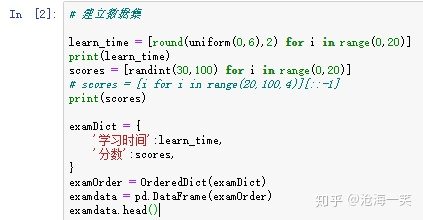这里我们我没用用真实的数据，使用的随机生成的数据进行讲解，大部分产生的数据都是不相关的。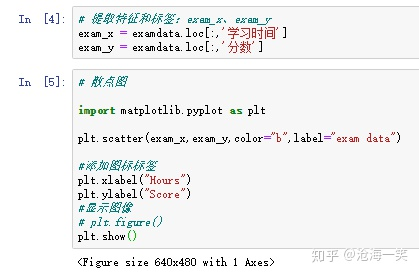提取特征，这部分。我在我在pycharm 中执行是可以显示现实图片的，这里只是给出了对象。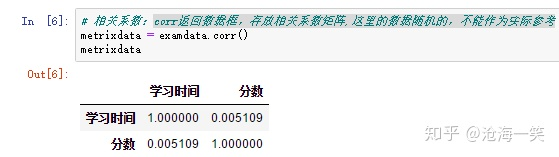相关系数，我这里显示的相关系数是0.005109，这里如果我再刷新一次就是负数，基本是不相关的，这里不能用线性相关来解释。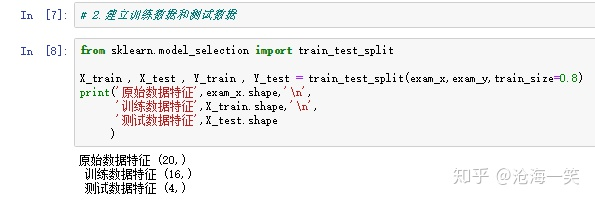这里的测试数据占用0.2，训练数据占用0.8，这个值得规定是train_testsplit中的train_size决定的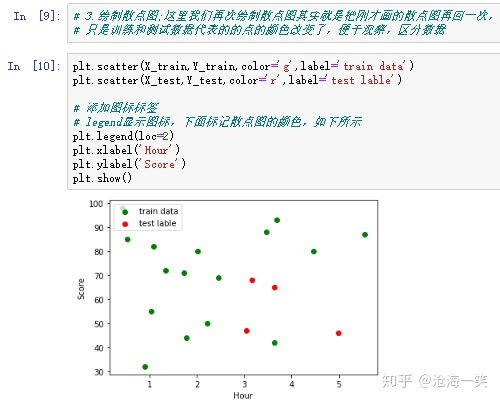这一步只是为了展示训练数据和测试数据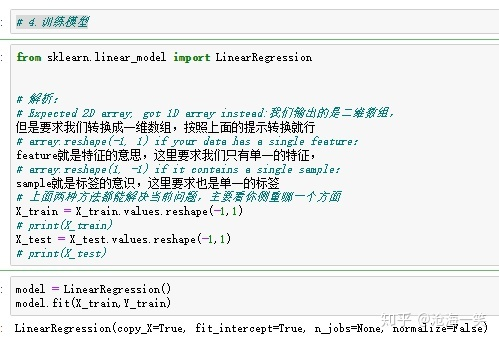训练模型，这一步我们需要将数组转换类型，如果不转换，直接操作LinearRegression()这个函数，我们会得到下面的错误：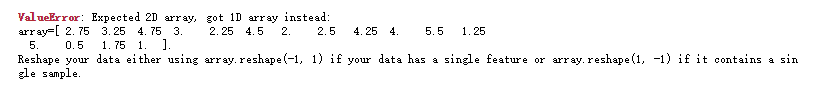其实解析这个错误看他的报错就行，至于怎么解开这个谜题，上面一张图片我已经给出答案。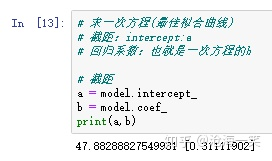这里我们绘制直线得出的截距和回归系数这个在绘图中是用不到的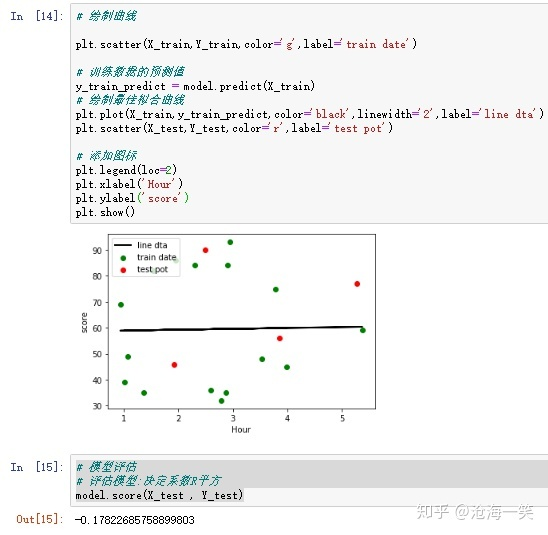这里最后再画一次其实就是对照一下，求决定系数R平方，这里开方了，得出的结果，我想大家看懂了吧，这个数这么小，而且是负数，说明这条回归线不能描述这组数据的准确性。

好了，这次讲解，主要是对简单的线性回归属性的回顾，操作的代码很少。

展开全文• 1.需求引入 有联系的事物之间存在着特定的关系。将事物抽象为变量，即变量之间存在着特定的关系。回归（regression）方法就是建立变量之间相互关系模型的数学方法。...2.一元回归 2.1一元线性回归 一机器学习 人工智能 大数据
• 一元回归公式5.估计的回归公式6.最小二乘法得到回归线应该穿过中心点7.预测值8.误差项9.斜率公式10.截距公式11. 决定系数R**212.线性关系检验13.相关系数检验14.残差15.被调整的R平方(The Adjusted R2 Value)16.回归...python回归代码
• 该问题是典型的一元回归问题，但 先要确定是线性还是非线性 ，然后就可以利用对应的回归方法建立他们之间的回归模型了，具体实现的 MATLAB 代码如下： （1）输入数据 clc, clear all, close all x=[23.80,...
• 一元线性回归spss处理方法，可以了解SPSS对线性回归的处理内容线性回归
• ab(x) 4、讨论之前的作业：用matlab计算一元回归系数并作图。 M文件 function [a,b]=yiyuanhuigui(x,y) pingjunshu_x=mean(x); pingjunshu_y=mean(y); b=[x*y'- pingjunshu_x*sum(y)]/[sum(x.^2)- pingjunshu_x*sum...
• 此文作为我的数模开篇，主要讲述建模的大概历程和一元回归分析类问题的解决方案。 一、常见统计建模方法 注：参照汪晓银老师的讲义，如有侵权，联系作者 1.预测与预报 灰色预测模型 回归分析预测 微分方程预测 ...
• 讨论幂函数、指数函数、双曲函数及S型曲线通过换元进行线性回归时，所满足的最小二乘条件，指出换元线性化回归存在的问题，提出了改进措施，并给出幂函数和双曲函数回归的实际算例。理论分析与实验表明，换元后的因...
• 回归分析概述及一元回归模型参数估计PPT课件.pptx
• 一元回归分析以及二元回归分析的详细讲解与分析，以及回归分析在matlab中的应用
• 一元回归分析100428PPT学习教案.pptx...